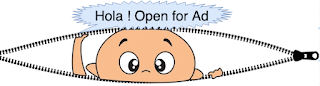## Latest Posts

Level order traversal is one of common interview question and it creates foundation of various other problems.Displaying nodes in reverse order is one of the variation of level order traversal.
In order to visit tree nodes in reverse order (level order fashion bottom to top), we need to store dequeued nodes from queue into a stack and iterate stack to display nodes in reverse order.Consider following tree and its level order traversal node sequence.

Reverse level order traversal nodes sequence :-   56 78 32 98 11 43 12 27 23 18 12
Algorithm:-
1. Do check for empty tree(root is not null), enqueue root into queue.
2. Loop until queue is not empty
Dequeue node from queue and push it into stack. Check for left and right child, if exist enqueues
them into queue, right child followed by left child.
3. Repeat step 2.
3. Exit loop when queue becomes empty
Sample code for iterative reverse level order traversal :-
```public void reverseIterativelevelOrderTraversal(Node root) {
/* java.util.ArrayDeque and java.util.Stack */
Queue<Node> queue = new ArrayDeque<>();
Stack<Node> stack = new Stack<>();
if (root != null) {
while (!queue.isEmpty()) {
/* Dequeue form queue */
root = queue.remove();
stack.push(root);
Node lc = root.leftChild;
Node rc = root.rightChild;
/* Enqueue into queue first right followed by left child */
if (rc != null) {
}
if (lc != null) {
}
}
}
while (!stack.isEmpty()) {
System.out.print(stack.pop().data + " ");
}
}```
Explanation:- Extra space of order O(n) is used as stack and on each dequeue operation stack is filled. Right child followed by left child enqueued into queue because we need to pop left node first from stack and stack follows LIFO property.

#### Complete sample program for reverse level order traversal

 `package com.devinline.trees;import java.util.ArrayDeque;import java.util.Queue;import java.util.Stack;public class ReverseLeveLOrderTraversal {  public void reverseIterativelevelOrderTraversal(Node root) {    /* java.util.ArrayDeque and java.util.Stack */    Queue queue = new ArrayDeque<>();    Stack stack = new Stack<>();    if (root != null) {      queue.add(root);      while (!queue.isEmpty()) {        /* Dequeue form queue */        root = queue.remove();        stack.push(root);        Node lc = root.leftChild;        Node rc = root.rightChild;        /* Enqueue into queue first right followed by left child */        if (rc != null) {          queue.add(rc);        }        if (lc != null) {          queue.add(lc);        }      }    }    while (!stack.isEmpty()) {      System.out.print(stack.pop().data + " ");    }  }  public static void main(String[] args) {    ReverseLeveLOrderTraversal rlo = new ReverseLeveLOrderTraversal();    Node root = rlo.new BinaryTree().createTree();    System.out.print("Reverse level order traversal(bottom to top) : ");    rlo.reverseIterativelevelOrderTraversal(root);  }  class BinaryTree {    Node root;    public BinaryTree() {      root = null;    }    public Node createTree() {      if (root == null) {        root = new Node(12);      }      root.setLeftChild(new Node(23));      root.setRightChild(new Node(18));      root.getLeftChild().setLeftChild(new Node(11));      root.getLeftChild().setRightChild(new Node(43));      root.getRightChild().setRightChild(new Node(27));      root.getRightChild().setLeftChild(new Node(12));      root.getLeftChild().getLeftChild().setLeftChild(new Node(56));      root.getLeftChild().getLeftChild().setRightChild(new Node(78));      root.getRightChild().getLeftChild().setRightChild(new Node(98));      root.getRightChild().getLeftChild().setLeftChild(new Node(32));      return root;    }  }  class Node {    private int data;    private Node leftChild;    private Node rightChild;    public Node(int data) {      this.data = data;      leftChild = null;      rightChild = null;    }    public int getData() {      return data;    }    public void setData(int data) {      this.data = data;    }    public Node getLeftChild() {      return leftChild;    }    public void setLeftChild(Node leftChild) {      this.leftChild = leftChild;    }    public Node getRightChild() {      return rightChild;    }    public void setRightChild(Node rightChild) {      this.rightChild = rightChild;    }  }}`
================Sample output==============
Reverse level order traversal(bottom to top) : 56 78 32 98 11 43 12 27 23 18 12
=========================================

#### 1 comment:

1.